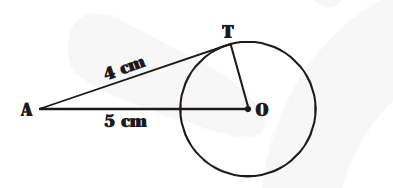# The length of a tangent from a point A at a distance 5 cm from the centre of the circle is 4 cm.

Question:

The length of a tangent from a point A at a distance 5 cm from the centre of the circle is 4 cm. Find the radius of the circle.

Solution:

The tangent to a circle is perpendicular to the radius through the point of contact.

$\therefore \quad \angle \mathrm{OTA}=90^{\circ}$

Now, in the right $\triangle \mathrm{OTA}$, we have :

$\mathrm{OA}^{2}=\mathrm{OT}^{2}+\mathrm{AT}^{2}$      [Pythagoras theorem]$\Rightarrow 5^{2}=\mathrm{OT}^{2}+4^{2}$

$\Rightarrow \mathrm{OT}^{2}=5^{2}-4^{2}$

$\Rightarrow \mathrm{OT}^{2}=(5-4)(5+4)$

$\Rightarrow \mathrm{OT}^{2}=1 \times 9=9=3^{2}$

$\Rightarrow \mathrm{OT}=3$

Thus, the radius of the circle is $3 \mathrm{~cm}$.